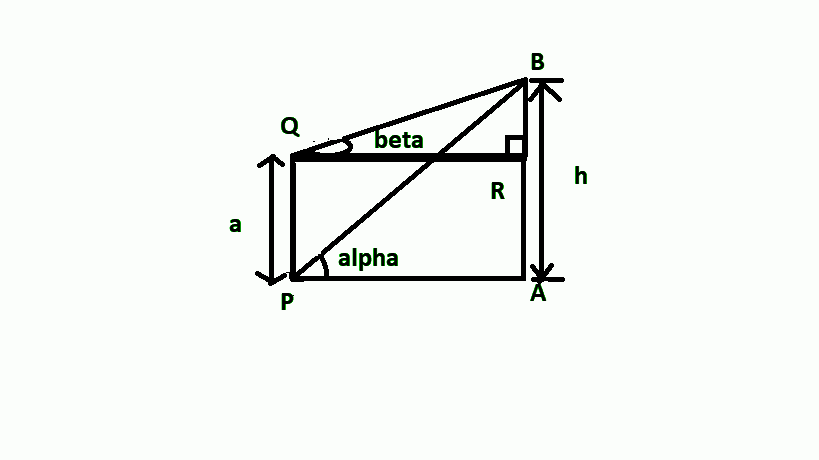QUESTION

# PQ is a post of height $a$, and A is a tower at some distance; $\alpha$and $\beta$ are the angles of elevation of B, the top of tower, at P and Q respectively. The height of the tower is${\text{A}}{\text{. }}\dfrac{{a\sin \alpha \cos \beta }}{{\sin (\alpha - \beta )}}${\text{B}}{\text{. }}\dfrac{{a\cos \alpha \cos \beta }}{{\sin (\alpha - \beta )}}${\text{C}}{\text{. }}\dfrac{{a\sin \alpha \sin \beta }}{{\sin (\alpha - \beta )}}$${\text{D}}{\text{.}}$ None of these

Hint: Draw the figure according to the information provided in the question and they analyse it to solve the question.

According to the question, PQ is a post of height a, and AB is the tower.
Let us assume the height of the tower be h.
The figure for the above question is shown below-From the figure we can see,
PQ = AR = a.
Therefore, we can also say that, BR = AB -AR – (1)
Now, we know, AB = h (assume)
Therefore, substituting AB = h and AR = a in equation (1), we get-
$BR = h - a - (2)$
Now, in right angled triangle BAP,
$\tan \alpha = \dfrac{{AB}}{{AP}} \\ \therefore \tan \alpha = \dfrac{h}{{AP}} \\ \Rightarrow AP = \dfrac{h}{{\tan \alpha }} - (3) \\$

Also, in right angle triangle BRQ,
$\tan \beta = \dfrac{{BR}}{{QR}} \\ \therefore \tan \beta = \dfrac{{h - a}}{{AP}}[\because AP = RQ] \\ \Rightarrow AP = \dfrac{{h - a}}{{\tan \beta }} - (4) \\$

From equation (3) and (4), we can say,
$\dfrac{{h - a}}{{\tan \beta }} = \dfrac{h}{{\tan \alpha }} \\ \Rightarrow h\tan \alpha - a\tan \alpha = h\tan \beta \\ \Rightarrow h(\tan \alpha - \tan \beta ) = a\tan \alpha \\ \Rightarrow h = \dfrac{{a\tan \alpha }}{{\tan \alpha - \tan \beta }} \\$

We can write, $\tan \alpha = \dfrac{{\sin \alpha }}{{\cos \alpha }},\tan \beta = \dfrac{{\sin \beta }}{{\cos \beta }}$

So, h will be equal to, $h = \dfrac{{a\dfrac{{\sin \alpha }}{{\cos \alpha }}}}{{\dfrac{{\sin \alpha }}{{\cos \alpha }} - \dfrac{{\sin \beta }}{{\cos \beta }}}} = a\dfrac{{\sin \alpha }}{{\cos \alpha }} \times \dfrac{{\cos \alpha \cos \beta }}{{\sin \alpha \cos \beta - \cos \alpha \sin \beta }} \\ \Rightarrow h = \dfrac{{a\sin \alpha \cos \beta }}{{\sin (\alpha - \beta )}} \\$

Hence, the height of the tower is, $h = \dfrac{{a\sin \alpha \cos \beta }}{{\sin (\alpha - \beta )}}$.
Therefore, the correct option is A.

Note- Whenever such types of questions appear, always write the information given in the question, and using that make a figure, find the value of the tangent of angle $\alpha ,\beta$, and then solve further by making required substitutions.